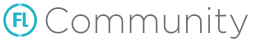Does not accept var inside MySQL SELECT

I use my SQL db for storing data and updating it works but selecting from it returns null.

To save text field text into the db in specific column based on the String variable works but not getting the data from it.

So I have nameString var that is set based on the action to a different name. The name value of the string matches a column name in the DB file.

So when myString is set to name “John”, it will save into this column and it works:

var nameString : String;
var slot1 : String;

slot1 = slot1TextField.text

let’s say slot1 = “It works”;
nameString = “John”;

`myDB.request(" UPDATE myDBTable SET '" + myDB.escape('\$nameString') + "' = '" + myDB.escape('\$slot1') + "' WHERE _rowid_ = 1 ");`

This saves with success the slot1 text in the DB at the column that nameString equals to.

The opposite, loading the data from the DB returns Null when trying to get the text:

``````public function whatevert()

var myDB = Sqlite.open('db/myDB.db');

var myNewVar = myDB("SELECT '" + myDB.escape('\$nameString') + "' FROM myDBTable WHERE _rowid_ = 1 ");
``````

Doesn’t like t he variable there?

if tracing it it detects nameString as it displays in the logs correct column name:

Log:
{ cache => { h => [{ ‘John’ => John },null], q => [{ ‘John’ => John },null], length => 1 }, r => #abstract }

returns null when trying to display the text, but if in the MYSQL I typed John instead of myDB.escape(’\$nameString’) it works

Code I use for displaying the DB value in a field after SELECT from …

``````	for (char in myNewVar)
{
var displayNewText = char.nameString;
myNewVarTextField = new TextField();
myNewVarTextField.background = false;
myNewVarTextField.border = false;
myNewVarTextField.multiline = false;
myNewVarTextField.selectable = false;
myNewVarTextField.text = '\$displayNewText';
myNewVarTextField.textColor = 0xFFFFFF;
}
``````

if I replaced myString with e.g John like "SELECT John FROM myDBTable or above char.John , only then it would not return null but the actual value “it works”

Not sure if it’s just a typo, but…
you do `UPDATE ... WHERE rowid = 1`
then `SELECT ... WHERE _rowid_ = 1`
…which is not the same field nameNo this is the forum formatting “_” probably makes it italic.`_rowid_`

is the internal ID row of any table but it works only if SELECT John instead of SELECT nameString(var). Updated the OP to not mislead

Does it work if you do this ?

``````	var myNewVar = myDB.request("SELECT " + myDB.escape(nameString) + " FROM myDBTable WHERE rowid = 1 ");
``````

`var myNewVar = myDB.request("SELECT '" + myDB.escape('\$nameString') + "' FROM myDBTable WHERE rowid = 1 ");`

I omitted the .request in my first post when posting but here is the full line, it fails to read the variable within the SQL command later on. Strange how it works for reading the var to store into the DB but not for loading from it.

Ok I edited my post as well, so does it work now ?
Because I’m not sure how is the ‘\$variable’ processed.

I tried this method but it still gives me null`var myNewVar = myDB.request("SELECT " + myDB.escape(nameString) + " FROM myDBTable WHERE _rowid_ = 1 ");`

Well there is some progress I posted in my OP that the log of tracing myNewVar shows:

`hx:389: { cache => { h => [{ 'John' => John },null], q => [{ 'John' => John },null], length => 1 }, r => #abstract }`

And with your method it shows the actual text near the Name
`hx:389: { cache => { h => [{ John => It works },null], q => [{ John => It works },null], length => 1 }, r => #abstract }`

Why does it not display it in text field I wonder in such case how should the text get it? It does get the value I think, since before that ‘It works’ was null instead

That’s because myDB.request returns a Resultset ( https://api.haxe.org/sys/db/ResultSet.html ).
So you have to do :

``````var rs = myDB.request("SELECT " + myDB.escape(nameString) + " FROM myDBTable WHERE rowid = 1");
var myNewVar = rs.getResult(0);
``````
1 Like

Ah thanks a lot!

Yes that worked and since the getResult made a clean string then in my TextField I omit the For loop and remove the var displayNewText = char.nameString;and instead my ‘\$displayNewText’ becomes `myNewVarTextField.text = '\$myNewVar ';`

You can also just do : `myNewVarTextField.text = myNewVar;`Indeed, it only existed because it was accessing the name from the DB for something I used in the past, but the above way is better and more correct thanks